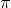The unit of current, the ampere, is defined in terms of the force between currents. Two 1.0-meter-long sections of very long wires a distanc

Question

The unit of current, the ampere, is defined in terms of the force between currents. Two 1.0-meter-long sections of very long wires a distance 2.0m apart each carry a current of 1.0 A

What is the force between them? (If the force between two actual wires has this value, the current is defined to be exactly 1 A.)

in progress 0
5 months 2021-08-10T20:09:34+00:00 2 Answers 12 views 0

Explanation: The force per unit length between both current carrying conductors is defined by the formulae below

F/L = u ×I1×I2/ 2πr

Where F = Force on both conductors,

L = length of both conductors = 1m,

I1 = I2 = 1A = current on both conductors,

r = 2m = distance separating conductors

u = permeability of free space = 1.256×10^-6 mkg/s²A²

By substituting the parameters, we have that

F/ 1 = (1.256×10^-6 ×1 ×1) / 2× 3.142 ×2

F/ 1 = 1.256×10^-6/ 12.566

F = 1.256×10^-6 / 12.566

F = 0.1×10^-6

F = 1×10^-5 N

Explanation:

Let the force on both conductors be F;

F=(U * I1 *I2 *k )/2d ………1

U=4X10^-7

I1=1.0A

I2=1.0A

k=1.0m

d=2.0m

Substituting , we have

F=(4X10^-7x1x1x1)/(2x2)

F=1×10^-7N